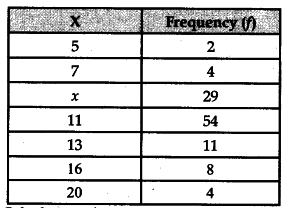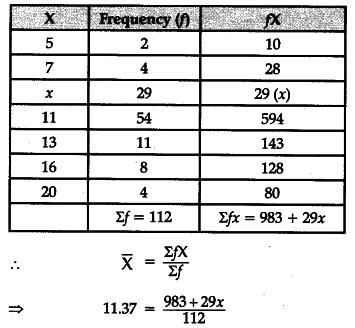# Find out the missing item x of the following,

Find out the missing item x of the following, where Arithmetic Mean (\bar { X } ) is 11.37.Calculation of Missing Item=> 1273.44 = 983 + 29x
=> x = 10.01 = 10
Hence, the missing value is 10.